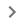# Use equations in a document

Want to get more out of Google Docs for work or school? Sign up for a free Google Workspace trial.

You can insert mathematical equations into your documents.

## Insert an equation

1. Open a document in Google Docs.
2. Click where you want to put the equation.
3. Click InsertEquation.
4. Select the symbols you want to add from one of these menus:
• Greek letters
• Miscellaneous operations
• Relations
• Math operators
• Arrows
5. Add numbers or substitute variables in the box.

To add another equation box, click New equation.

To show or hide the equation options, click ViewShow equation toolbar.

## Use equation shortcuts

You can type "\" followed by the name of a symbol and a space in an equation to insert that symbol. For example, \alpha will insert 𝞪.

To type superscripts or subscripts, type "\", then press Shift + 6 or Shift + -.

Was this helpful?
How can we improve it?